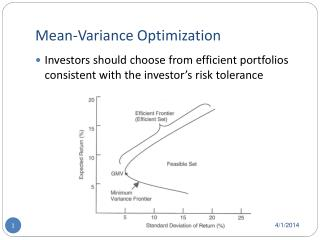DownloadDownload PresentationMean-Variance Optimization

# Mean-Variance Optimization

Télécharger la présentation## Mean-Variance Optimization

- - - - - - - - - - - - - - - - - - - - - - - - - - - E N D - - - - - - - - - - - - - - - - - - - - - - - - - - -
##### Presentation Transcript

1. Mean-Variance Optimization • Investors should choose from efficient portfolios consistent with the investor’s risk tolerance

2. Unconstrained Mean-Variance Optimization • Weights can take on any value (positive or negative), only constraint is that the weights sum to one • Black (1972) provided a short cut for finding minimum variance portfolios: • Asset weights of any minimum variance portfolio is a linear combination of the asset weights of any other two minimum variance portfolios (Black’s Two-Fund Theorem) • Mathematical proof provided in the 1972 paper

3. Unconstrained Mean-Variance Optimization Numerical example • Suppose we have three asset classes: equities (S), fixed income (B), and real estate(RE) • Composition of the minimum variance portfolio with E(r) = 10% is (70%, 20%, 10%) • Composition of the minimum variance portfolio with E(r) = 8% is (50%, 30%, 20%) • What is the composition of the minimum variance portfolio with E(r) = 9.5%?

4. Constrained M-V Optimization • Minimum asset class weight = 0 • Most relevant for strategic asset allocation • May short assets within a class, but not the entire class • To generate the efficient frontier, use the corner portfolio theorem of Markowitz (1959, 1987) • There are infinitely many efficient portfolios, only need a limited number of “corner portfolios” to identify them all • Corner portfolios: They are located when an asset class is either added or dropped along the efficient frontier (i.e., weight going from zero to strictly positive or from strictly positive to zero)

5. Constrained M-V OptimizationNumerical Example

6. Constrained M-V OptimizationCorner Portfolios GMV portfolio

7. Constrained M-V Optimization

8. Constrained M-V Optimization • Every efficient portfolio is a linear combination of the two corner portfolios immediately adjacent to it (on either side of it). Thus, by locating all corner portfolios, you can generate the entire efficient frontier • Markowitz (1959, 1987 – two books) provides the “critical line algorithm” to do this • Our textbook calls this the “Corner Portfolio Theorem”: • The asset weights of any minimum variance portfolio are a (positive) linear combination of the weights of the two adjacent corner portfolios that bracket it in terms of E(r)

9. Constrained M-V OptimizationNumerical Example • Find the composition of the mean-variance efficient portfolio E(r) = 8% • Which two corner portfolios does it lie in between? • Solve for the weights: • UK equities = ? • Ex-UK equities = ? • Intermediate bonds = ? • Long term bonds = ? • International bonds = ? • Real estate = ?

10. Constrained M-V Optimization • Variance of the 8% E(r) portfolio • Use the variance formula (sum of the variance-covariance matrix bordered by the weights) • In this case, there are four assets

11. Re-sampled Efficient Frontier Optimization • Mean variance optimization treats the inputs as population parameters • But they are only sample estimates • Estimation errors of the inputs will distort the optimization results • Most important input in MV optimization is E(r) • Ziemba (2003) show that estimation error in E(r) is 10 times as important as estimation error in , and 20 times as important as estimation error in 

12. Re-sampled Efficient Frontier Optimization • Re-sampling: Take several efficient portfolio simulations using different parameters for E(r), , and  as sensitivity analysis • For each level of E(r), average the weights of each asset class from different efficient portfolios (that were estimated using different inputs) • These can then be integrated into a re-sampled efficient frontier • Re-sampled efficient frontier tends to be more diversified and more stable over time

13. Monte-Carlo Simulation in Asset Allocation • Most likely application: • Given an existing asset allocation, calculate terminal wealth using random draws from historical distributions of returns • Provides information concerning the range of possible results and the relative likelihood of each (e.g., “80% of the times, will have terminal wealth greater than \$1 million, given current portfolio”)

14. Experience Based Approaches to Asset Allocation • Rely on tradition, experience and/or rules of thumb • Inexpensive to implement • 60/40 stock bond allocation as neutral starting point • Allocation to bonds increases with risk aversion • Allocation to stocks increases with time horizon • Allocation to equities = 100 - age

15. Strategic Asset Allocation for Defined Benefit Plans • Must maintain liquidity to pay current benefits • Asset/Liability Management used to control: • Shortfall risk • Volatility of pension surplus • Asset-only management seeks to minimize standard deviation relative to required return (e.g., 6%)

16. Rest of the chapter • Ideas for project • Contribution to risk by different asset classes (rows of the Var-Cov matrix)

17. New ways of thinking about diversification • Allocating capital across asset classes and investment styles represents superficial diversification if payoffs are exposed to the same set of risk factors. • Diversify across underlying risk factors • E.g., Bradley Jones, Rethinking Portfolio Construction and Risk Management: A Third Generation in Asset Allocation, 2012 (presentation available on the web) • 1st generation: 60/40 • 2nd generation: more asset classes

18. New ways of thinking about diversification • Equity risk premium prevails only in “normal” markets • Diversify across different risk exposures, such as: • interest rate risk • commodity risk • style risk premia, such as value, momentum and volatility • liquidity risk • sovereign risk